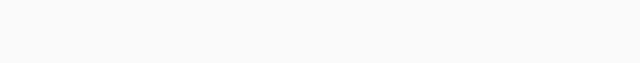# Highest Product | InterviewBit | Solution Explained

I explain the solution to Highest Product on InterviewBit using visuals in simple words.

## 0. Highest Product: Problem Discussion

### 0.0. IO Format

• input:
• array of N integers
• output:
• Highest product possible by multiplying 3 elements.
• constraints:
• 3 <= N <= 5e5

### 0.1. Examples

Input: [1, 2, 3, 4]

Output: 2 * 3 * 4 = 24

Input: [0, -1, 10, 7, 5]

Output: 5 * 7 * 10 = 350

## 1. Highest Product: Observations & Logic

### 1.0. Intuitions

At first thought, it looks like we can simply multiply the biggest 3 elements. This works well in the case [1,2,3,4] where the answer is just 2*3*4 = 24. This also works in the case [0,-1,10,5,7] giving us 5*7*10. So, this is it?

### 1.1. Disproving

Whenever we have a hypothesis for a solution, it is always a good idea to disprove it. If you can not find a edge-case, then the idea might be the correct one. However, if you can disprove it, don’t be sad! You just found another test case to work upon and build your observations.

Let’s take a look at some more examples:Highest Product: test case that validates the initial thoughts

This case works out perfectly. So, good going! However, what about the case below?Highest Product: test case that invalidates the initial thoughts

We have the wrong answer! Why? Notice how we can multiply -5 and -2 on the left to give 10 (a positive value!) and then multiply it by 5 on the right to get the correct answer of 50.

### 1.2. The correct logic

The above test case clearly shows that the last two negative values can be combined with a positive and it might give a better result. It is thus evident that in some cases, we have to use the logic of 2 negative and 1 positive and in some cases, we simply pick the first 3 values. Which is correct?

Well, it is impossible to know without testing both out. So, what if we simply take the max of both? Basically, we are looking at either:

• case1: the highest 3 elements (the 3 on the right)
• case2: the 2 lowest elements (2 negative values) and the highest positive value on the right.
• ans = max(case1, case2)Highest Product: case1 and case2 combined

All right! Let’s implement it.

## 2. Highest Product: Optimized Implementation

### 2.0. Code

```class Solution:
# @param A : list of integers
# @return an integer
def maxp3(self, A):
A = sorted(A)

hi3 = A[-1] * A[-2] * A[-3]
lo2hi1 = A * A * A[-1]

return max(hi3, lo2hi1)
```

### 2.1. Complexity Analysis

• Time: `O(NlogN)` to sort the array.
• Space: `O(1)`, to store the hi3 and lo2hi1 variables.
##### Tanishq ChaudharyProducing high-quality intuitive explanations of interview problems. Currently covering LeetCode and InterviewBit.

### Recommended Posts

##### Gas Station | InterviewBit | Solution Explained

June 13, 2022

##### Majority Element | InterviewBit | Solution Explained

June 13, 2022

##### Assign Mice to Holes | InterviewBit | Solution Explained

June 13, 2022

1.Srishti Chopra :July 23, 2022 at 11:29 am
2.Makarand :August 16, 2022 at 11:06 am